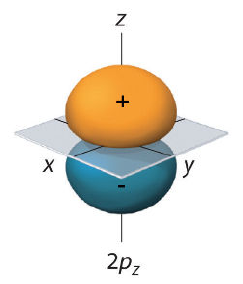Study Guides (400,000)
US (230,000)
UC-Irvine (3,000)
CHEM (100)
Quiz

# CHEM 132B Study Guide - Quiz Guide: Wave Function, Spherical Harmonics, Momentum OperatorExam

Department
Chemistry
Course Code
CHEM 132B
Professor
Eric Potma
Study Guide
Quiz

This preview shows page 1. to view the full 5 pages of the document.Chem 132B Quiz 2 Key
Version A
Problem 1 (16 points)
Consider the 2pzorbital shown in the image above. Indicate whether the fol-
lowing statements are True or False. (4 points each)
a) The electron is located on either side of the nodal plane, and not on both
sides simultaneously. False
b) There is a nonzero chance of ﬁnding the electron outside of the shaded
volume. True
c) The sign reversal along zcorresponds to a spin ﬂip of the electron. False
d) It is less likely to ﬁnd the electron on the side with negative amplitude
than on the side with positive amplitude. False
Problem 2 (16 points)
Consider the wavefunction of an atomic system. Indicate whether the following
statements are True or False. (4 points each)
a) If the spin state is symmetric, then the orbital wavefunction must be anti-
symmetric. True
b) If the spin state is in a singlet state, then the orbital wavefunction is
symmetric. True
c) If the spin state is in a triplet state, then the electrons must occupy
diﬀerent orbital wavefunctions. True
d) If the spin state is anti-symmetric, then the electrons must occupy the
same orbital wavefunction. False
1

Unlock to view full version

Only page 1 are available for preview. Some parts have been intentionally blurred.Problem 3 (34 points)
The wavefunctions of the hydrogen atom can be written as |Ψn,l,mli=|n, l, mli
a) The atom is in de excited state |2,1,1i. Give the full expression for the
wavefunction in terms of coordinates (r, θ, φ). (8 points)
Ψ2,1,1(r, θ, φ) = 3
8π1/21
24 1
a05/2
rer/2a0sin θ e
This can be simpliﬁed to:
Ψ2,1,1(r, θ, φ) = 1
64π1/21
a05/2
rer/2a0sin θ e
b) How many angular planar nodes does |2,1,1ihave? (8 points)
One angular node is found at θ= 0, which is the same plane deﬁned
by θ=π. No nodes along φ.
c) Consider b
l2|2,1,1i. What is the magnitude of orbital angular momen-
tum of this state, and what is its projection onto the z-axis?
(5+5=10 points)
b
l2|2,1,1i=l(l+ 1)¯h2|2,1,1i= 2¯h2|2,1,1i
Magnitude of orbital angular momentum is h.
Since b
lz|2,1,1i=ml¯h|2,1,1i=¯h|2,1,1i, projection is ¯h.
d) Can the atom be excited from |2,1,1ito |4,0,1i? Explain. (8 points)
Yes, ∆l=1, which is a valid transition, since allowed transition have
l=±1
2